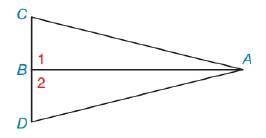Chapter 3.2, Problem 5E### Elementary Geometry for College St...

6th Edition
Daniel C. Alexander + 1 other
ISBN: 9781285195698

#### Solutions

Chapter
Section### Elementary Geometry for College St...

6th Edition
Daniel C. Alexander + 1 other
ISBN: 9781285195698
Textbook Problem
1 views

# In Exercise 5 to 12, plan and write the two-column proof for each problem. Given: ∠ 1 and ∠ 2 are right ∠ s C A ¯ ≅ D A ¯Prove: Δ A B C ≅ Δ A B D

To determine

To prove:

The given statement.

Explanation

Given:

The following figure shows the given diagram.

Given that,

1 and 2 are right s and CA¯DA¯.

Two right triangles will be congruent if the hypotenuse and corresponding lag are equal in both triangles.

Approach:

The completed proof is shown in the following table.

 Proof Statements Reasons 1. ∠1 and ∠2 are right ∠s 1. Given 2. CA¯≅DA¯ 2. Given 3. ∠1=90° 3. Definition of right angle. 4. ∠2=90° 4. Definition of right angle. 5. ∠1≅∠2 5. Definition of congruent triangles. 6. AB¯≅AB¯ 6

### Still sussing out bartleby?

Check out a sample textbook solution.

See a sample solution

#### The Solution to Your Study Problems

Bartleby provides explanations to thousands of textbook problems written by our experts, many with advanced degrees!

Get Started

#### Find more solutions based on key concepts